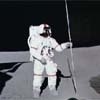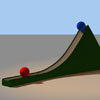#### You may also like### Lunar Leaper

Gravity on the Moon is about 1/6th that on the Earth. A pole-vaulter 2 metres tall can clear a 5 metres pole on the Earth. How high a pole could he clear on the Moon?### Which Twin Is Older?

A simplified account of special relativity and the twins paradox.### Whoosh

A ball whooshes down a slide and hits another ball which flies off the slide horizontally as a projectile. How far does it go?

# Cannon Balls

##### Age 16 to 18 ShortChallenge Level

We recieved solutions from Vladimir Radmanikov from South Island School, Samuel from Manor Park Community college and Laura from Millhaven school, who said the following:

If we take upwards as positive $x$ then this is motion under a constant downwards acceleration $a=-9.8$. We need to use the formula
$$x(t) = v(0)t+\frac{1}{2}at^2= v(0)t-4.9t^2\;.$$
We want to know the starting speed $v(0)$ to make it hit the ground $x=0$ in $1\mathrm{s}$.
So, we must solve
$$0 = v(0)\times 1 -4.9\times 1^2\;.$$
So, the ball must be shot up at $4.9\mathrm{ms}^{-1}.$

For other times we have
$$v(t) = 4.9t\;.$$
So, double the time requires double the speed and so on. So, $10\mathrm{s}$ travel time requires $49\mathrm{ms}^{-1}$ etc., as in this table

 Travel time $10\mathrm{s}$ $100\mathrm{s}$ $1000\mathrm{s}$ $100,000\mathrm{s}$ Speed $49\mathrm{ms}^{-1}$ $490\mathrm{ms}^{-1}$ $4.9\mathrm{kms}^{-1}$ $490\mathrm{kms}^{-1}$

Clearly the last one will go straight into space!

You used various method to find the higest point of the cannon ball, which occurred half way through the journey.

Samuel said

We need to work out when the ball stops moving to find the highest point each time. Differentiating the first equation gives us
$$v(t) = v(0) -9.8 t\;.$$
If $T$ is the time of maximum height then setting $v(T)=0$ gives $T = v(0)/9.8= t/2$. So it reaches the maximum height half way through the travel time in each case. Putting $t=5,50,500,500000$ into the first equation gives

 Travel time $10\mathrm{s}$ $100\mathrm{s}$ $1000\mathrm{s}$ $100,000\mathrm{s}$ Maximum height $122.5\mathrm{m}$ $12.25\mathrm{km}$ $1225\mathrm{km}$ $12250000\mathrm{km}$

Laura noted

By conservation of energy the cannon ball must hit the ground with the same speed as it left the ground, so the up and down parts of the journey are mirror images. So the maximum height is reached exactly half way through the journey. So, at times $5\mathrm{s}$, $50\mathrm{s}$, $500\mathrm{s}$, $50000\mathrm{s}$.

Vladimir made use of another form of the kinematic equations

To find the maximum height I can use this formula
$$v(t)^2 = v(0)^2 + 2ax(t)\;.$$
At the highest point $v(t)=0$, so
$$x_{max} =\frac{v(0)^2}{2\times 9.8}\;.$$

This method also gives the values in the previous table.

Vladimir though that, since the earths radius is $6000\mathrm{km}$ the cannon ball taking $1000\mathrm{s}$ was likely to return to earth, as this has a maximum height of $1225\mathrm{km}$, and was therefore still in range of gravity (even taking the decay of gravity into account). Laura noted that the escape velocity from earth is around $11\textrm{ km per second}$, so all but the final cannon ball would return to earth.

Well done to you all!